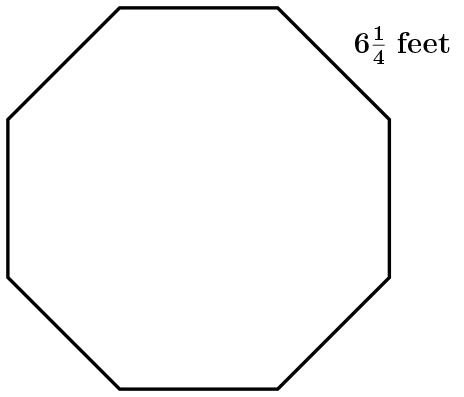# Polygon Area

Using our knowledge from the previous lesson of the properties of regular polygons to answer the following questions.

1. Draw in the appropriate amount of isosceles triangles to fill the regular octagon.1. Use the isosceles triangles to find the perimeter of the octagon.
2. Calculate the area of the octagon.

If a visual or further explanation is needed, the following link can help. It also offers a different look at how the formula can be developed.